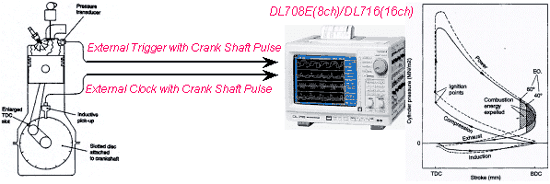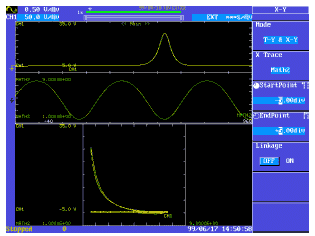# Simple Creation of Indicator Diagrams (P-V Diagram)

The characteristics of the cylinder pressure rise during the power stroke can be analyzed by the cylinder pressure on a piston stroke (Cylinder Volume) base indicator diagram (P-V Diagram). The combustion process can be broken into three phases or periods, (1)the delay period, (2)the rapid pressure rise period and (3)the after burning period. All of the pertinent data can be made available by using a dynamometer and a PC based spark ignition combustion analysis system. These systems can be expensive and difficult to set up. Using the Yokogawa DL708E/DL716 can simplify making the Indicator Diagram.Solutions
The Yokogawa Model DL708E 8-Channel/ DL716 16-Channel provide the following solutions for this application :

1. Unique Parameter "T" Is A User Define Math Function Providing Crank Angle Information
2. Knowing crank angle information can assist in getting cylinder volume. The T parameter in the user defined math function provides that information by using the crank shaft pulses.
3. User Define Math Function To Get Cylinder Volume Value To Write P-V Diagram
4. DL708E/DL716 user defined math function provides a calculation capability to obtain cylinder volume at each crank angle. Volume = P + S x (Q + R - SQR(Q x Q - (R cos q )x(R cos q )) + R sin q ) P:capacity of combustion chamber, S:sectional area of cylinder Q:length of con'rod, R:radius of crank, q :crank angle
5. Strain Module For Direct Input From Pressure Transducer
6. The Strain Module provides transducer excitation,signal conditioning and linear scaling with user assigned engineering units.The figure (left) is an example of drawing an Indicator Diagram. CH1 (yellow) is pressure signal of cylinder and CH2 (green) is cylinder volume curve by the above calculation in user define math.

Oben